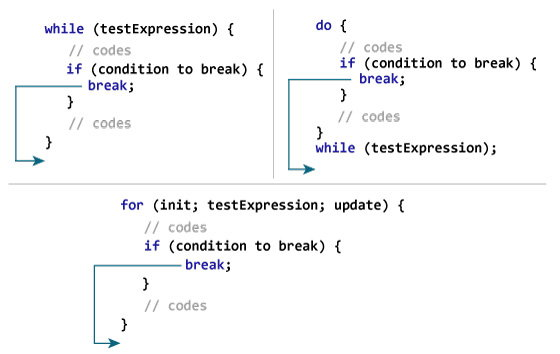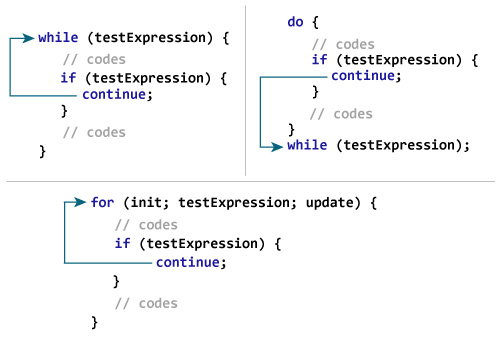# What is a break and continue in c

## C break

The break statement ends the loop immediately when it is encountered. Its syntax is:
```
`break;`

```
The break statement is almost always used with `if...else` statement inside the loop.

### How break statement works?### Example 1: break statement

```
`// Program to calculate the sum of a maximum of 10 numbers`
`// If a negative number is entered, the loop terminates`

`# include <stdio.h>`
`int main()`
`{`
`    int i;`
`    double number, sum = 0.0;`

`    for(i=1; i <= 10; ++i)`
`    {`
`        printf("Enter a n%d: ",i);`
`        scanf("%lf",&number);`

`        // If the user enters a negative number, the loop ends`
`        if(number < 0.0)`
`        {`
`            break;`
`        }`

`        sum += number; // sum = sum + number;`
`    }`

`    printf("Sum = %.2lf",sum);`
`    `
`    return 0;`
`}`

```
Output
```Enter a n1: 2.4
Enter a n2: 4.5
Enter a n3: 3.4
Enter a n4: -3
Sum = 10.30```

## C continue

The `continue` statement skips the current iteration of the loop and continues with the next iteration. Its syntax is:
```
`continue;`

```
The `continue` statement is almost always used with the `if...else` statement.

### How continue statement works?### Example 2: continue statement

```
`// Program to calculate the sum of a maximum of 10 numbers`
`// Negative numbers are skipped from the calculation`

`# include <stdio.h>`
`int main()`
`{`
`    int i;`
`    double number, sum = 0.0;`

`    for(i=1; i <= 10; ++i)`
`    {`
`        printf("Enter a n%d: ",i);`
`        scanf("%lf",&number);`

`        if(number < 0.0)`
`        {`
`            continue;`
`        }`

`        sum += number; // sum = sum + number;`
`    }`

`    printf("Sum = %.2lf",sum);`
`    `
`    return 0;`
`}`

```
Output
```Enter a n1: 1.1
Enter a n2: 2.2
Enter a n3: 5.5
Enter a n4: 4.4
Enter a n5: -3.4
Enter a n6: -45.5
Enter a n7: 34.5
Enter a n8: -4.2
Enter a n9: -1000
Enter a n10: 12
Sum = 59.70```